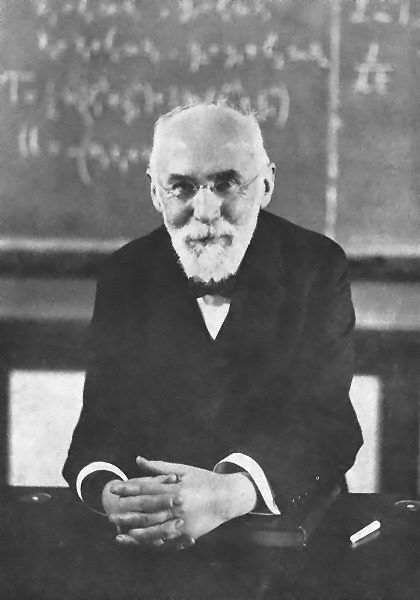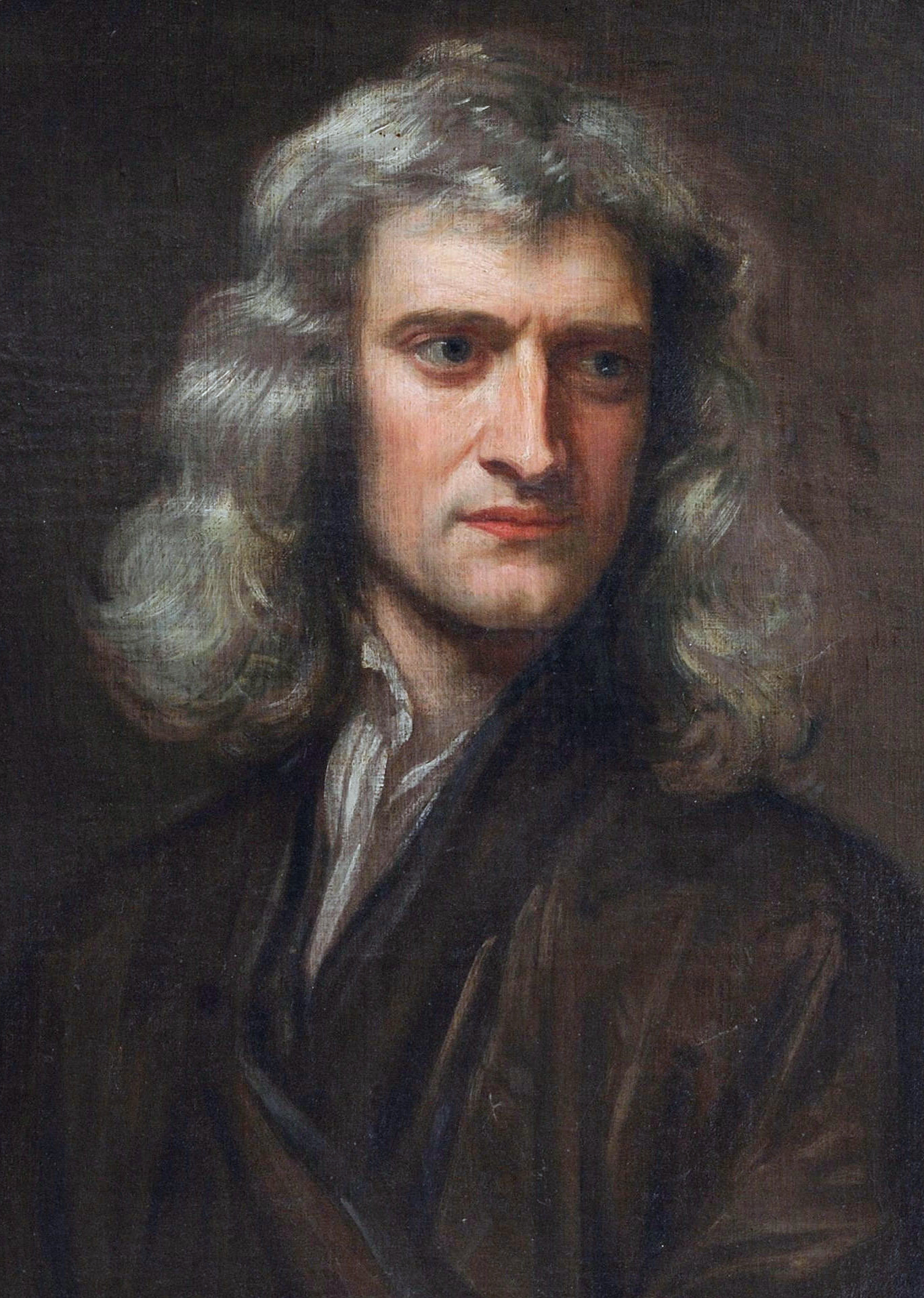## Famous physicists – A to Z

I was catching up with blog-posts accumulated on my reader during a vacation, when it occurred to me that there aren’t as many posts as one might expect during the A–to–Z challenge (it requires one to blog about a theme every day, except Sundays, throughout April). As Tara Sparling predicted, I appreciate that there weren’t hundreds of posts in my reader. Being still in a “vacation mood”, this post is a twist on the A–to–Z challenge – it presents one eminent physicist for every letter of the alphabet (for letters like “c” and “w” I chose from several names, while letters “q” and “x” were challenging).

 Ampère André-Marie Ampère (1775 – 1836). One of the founders of the science of classical electrodynamics.Bohr Niels Bohr (1885 – 1962). Based the understanding of the structure of the atom on quantum theory. Nobel Prize in Physics 1922.Curie Marie Curie (1867 – 1934). Made pioneering research on radioactivity. Nobel Prize in Physics 1903, Nobel Prize in Chemistry 1911.Dirac Paul Dirac (1902 – 1984). Formulated the Dirac equation, which gives a quantum relativistic description of the behavior of electrons and predicts the existence of their antiparticles – the positrons. Nobel Prize in Physics 1933.Einstein Albert Einstein (1879 – 1955). Formulated the theories of general and special relativity, and the theory of Brownian motion. Postulated the existence of quantum of light to explain the photoelectric effect. Nobel Prize in Physics 1921.Faraday Michael Faraday (1791 – 1867). Established the basis for the concept of the electromagnetic field in physics, discovered electromagnetic induction. Faraday’s discoveries made such an impact on the progress of science and of industry, that he is considered one of the most influential scientists in history.Galilei Galileo Galilei (1564– 1642). One of the giants of the scientific revolution during Renaissance. Galileo’s astronomical observations and his work on the motions of bodies were precursors of classical mechanics, relativity, modern astronomy.Heaviside Oliver Heaviside (1850 – 1925 ). Reformulated Maxwell’s equations into the form by which they have been known ever since, invented the Heaviside step function and employed it to model the current in an electrical circuit. His work changed telecommunications, mathematics and science.Ising Ernst Ising (1900 – 1998). Best known for Ising model – a mathematical model to calculate ferromagnetic interaction between spins. Today, Ising model is used to address problems in such diverse fields as neural networks, protein folding, biological membranes and social behavior. Joule James Prescott Joule (1818 – 1889). Discovered the relationship between heat to mechanical work. Joule’s measurements led to the law of conservation of energy (in a closed system, energy can be transformed from one form to another, but cannot be created or destroyed).Kepler Johannes Kepler (1571 – 1630). A key figure in the 17th century scientific revolution. Kepler’s laws of planetary motion introduced physics into astronomy (they were one of the foundations for Newton’s theory of gravitation).Lorentz Hendrik Lorentz (1853 – 1928). Theorized that the atoms consist of charged particles and that these charged particles’ oscillations are the source of light. Lorentz made fundamental contributions to the fields of electromagnetism, the electron theory, and relativity. Nobel Prize in Physics 1902.Maxwell James Clerk Maxwell (1831 – 1879). Formulated the classical theory of electromagnetic radiation. Maxwell’s equations bring together for the first time electricity, magnetism, and light as manifestations of the same phenomenon.Newton Isaac Newton (1642 – 1727). One of the most influential scientists of all time. In physics, he laid the foundations for classical mechanics, gravitation, and made seminal contributions to optics.Oersted Hans Christian Ørsted (1777 – 1851). Discovered that electric currents create magnetic fields. By confirming a direct relationship between electricity and magnetism, Oersted discovered electromagnetism. (Before his discovery, electricity and magnetism were thought of as two separate forces.)Planck Max Planck (1858 – 1947). An originator of the quantum theory. Discovered Planck’s constant – a fundamental physical constant upon which much of quantum theory is based. Nobel Prize in Physics 1918.Qurra Thābit ibn Qurra (826 – 901). Proposed a theory of motion in which both the upward and downward motions are caused by weight. In mechanics, he was a founder of statics. Rutherford Ernest Rutherford (1871 – 1937). Discovered that hydrogen nuclei was a new particle, which he dubbed the proton. Rutherford’s research, and work done under him as laboratory director, established the nuclear structure of the atom and the nature of radioactive decay as a nuclear process. Nobel Prize in Chemistry 1908.Schrödinger Erwin Schrödinger (1887 – 1961). Founder of wave quantum mechanics. Schrödinger equation describes how the wave function of the physical system changes with time. It “created a revolution in quantum mechanics and indeed of all physics and chemistry.” Nobel Prize in Physics 1933.Thomson J. J. Thomson (1856 – 1940). Discovered the electron. Nobel Prize for Physics 1906.Uhlenbeck George Uhlenbeck (1900 – 1988). Uhlenbeck and Goudsmit discovered the electron spin (an intrinsic angular momentum of the electron.) Volta Alessandro Volta (1745 – 1827). Invented the first modern electrical battery. Volta’s invention sparked other experiments which eventually led to the development of the field of electrochemistry.Wu Chien-Shiung Wu (1912 – 1997). Conducted the experiment which established that conservation of parity is violated by the weak interaction (the discovery earned the 1957 Nobel prize in physics for Wu’s theorists colleagues.)Xing Yi Xing (683 – 727). An astronomer and mathematician (and also a Buddhist monk) who studied the variations in the motions of celestial bodies. He found that stars move by themselves and are not fixed to celestial sphere.Yang Thomas Young (1773 – 1829). Established the wave theory of light through interference and diffraction experiments.Zeeman Pieter Zeeman (1865 – 1943). Discovered the splitting of spectral lines by a strong magnetic field, also known as Zeeman effect. Nobel Prize in Physics 1902.This entry was posted in physics and tagged , , , , . Bookmark the permalink.